# The water barrel

The water barrel weighs 122 kg. If we pour 75% of the water out of it, it will weigh 35 kg. What is the weight of the barrel?

s =  6 kg

### Step-by-step explanation:

s+v=122
s + v -0.75 v = 35

s+v=122
s + v -0.75•v = 35

s+v = 122
s+0.25v = 35

s = 6
v = 116

Our linear equations calculator calculates it.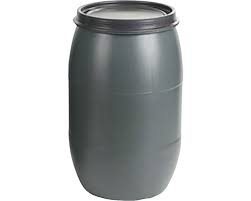Did you find an error or inaccuracy? Feel free to write us. Thank you!Tips to related online calculators
Do you have a system of equations and looking for calculator system of linear equations?
Tip: Our volume units converter will help you with the conversion of volume units.

## Related math problems and questions:

• Barrel 4Barrel of water weighs 63 kg. After off 75% water, the weight of the barrel with water is 21 kg. How many kg weigh empty barrel and how many kgs water in it?
• Barrel 3Barrel with water weights 118 kg. When we get off 75% of water, it weights 35 kg. How many kg has an empty barrel?
• Camel and water84% of the camel's weight is water. After drinking, its weight increased to 832 kg, and water accounted for 85% of its weight. How much did it weigh before drinking?
• Barrel with water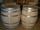The water barrel has a weight of 52 kg. When we pour a quarter of the water, the weight of the barrel is reduced to 40 kg. Determine the weight of the empty barrel.
• Barrel of oilBarrel of oil weighs 283 kg. When it mold 26% oil, weighed 216 kg. What is the mass of the empty barrel?
• Water containerContainer with water weighs 1.48 kg. When we cast 75% of water container of water weight 0.73 kg. How heavy is an empty container?
• Barrel with liquid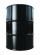Barrel with grain weight 297 kg. When it shed 48% of grain, ha weight 174 kg. What is the weight of an empty barrel?
• Container with water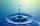The weight of a container with water is 2.48 kg. If the cast 75% water, the container with water has a weight of 0.98 kg. Determine the weight of the empty container. How much water was originally in the container?
• The barrel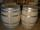The barrel is 135 cm high. If we fill it to a height of 60 cm and it weighs 163 kg. If we fill it to a height of 80 cm, it weighs 213 kg. How many kg weighs a full and how much empty barrel?
• WeightlifterWeightlifter lifted 75% of its weight. Determine how much weight lifted when he weighs 132 kg.
• Barrel 2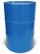Empty barrel weighs 7 kg. When it filled with water up to 45% height, weighs 60 kg. How heavy is barrel full of water?
• Three friendsDanica, Lenka, and Dalibor have 96 kg altogether. Lenka weighs 75% more than Dalibor, and Danica weighs 6 kg more than Dalibor. Determine the weight of Danice, Lenka, and Dalibor.
• Three piles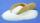100 kg of sugar we divide into three piles. The first pile is small. If we added to the second 2 kg of sugar, it would have 25% more sugar than the first pile. If we add 3 kg of sugar to the third pile it would have 20% more sugar than the 2nd pile. How m
• Electric cooker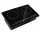During which time t does an electric cooker with power input P = 500 W and with efficiency n = 75% heat water with mass m = 2 kg and temperature t1 = 10°C to the boiling point (t2 = 100°C). The specific heat capacity of water is c = 4 180 J. Kg-1. K-1
• Base of percentages7% of the base is 35 kg. What is 39% of that base?
• Acid evaporationHow many kilograms of water do we have to evaporate from 100 kg of 32% acid to make it 80% concentration?
• Cargo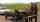The weight of the cargo car is 761 kg. The weight of the car is 23% of the total weight of car+cargo. What is the weight of the cargo load?